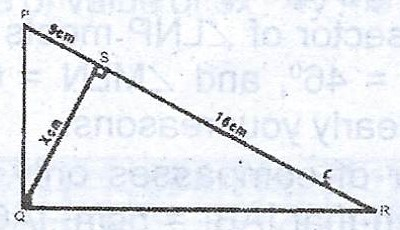# In the diagram, < PQR = < PSQ = 90°, |PS| = 9 cm, |SR| = 16 cm and |SQ| = x cm. (a) Find the value of x using a trigonometric ratio. (b) Calculate : (i) the...

Question 1In the diagram, < PQR = < PSQ = 90°, |PS| = 9 cm, |SR| = 16 cm and |SQ| = x cm.

(a) Find the value of x using a trigonometric ratio.

(b) Calculate : (i) the size of < QRS to the nearest degree; (ii) |PQ|.